# Ohm’s Law

## Chapter 2 - Basic Concepts and Test Equipment

PARTS AND MATERIALS

• Calculator (or pencil and paper for doing arithmetic)
• 6-volt battery
• Assortment of resistors between 1 KΩ and 100 kΩ in value

I’m purposely restricting the resistance values between 1 kΩ and 100 kΩ for the sake of obtaining accurate voltage and current readings with your meter.

With very low resistance values, the internal resistance of the ammeter has a significant impact on measurement accuracy.

Very high resistance values can cause problems for voltage measurement, the internal resistance of the voltmeter substantially changing circuit resistance when it is connected in parallel with a high-value resistor.

At the recommended resistance values, there will still be a small amount of measurement error due to the “impact” of the meter, but not enough to cause serious disagreement with calculated values.

CROSS-REFERENCES

Lessons In Electric Circuits, Volume 1, chapter 2: “Ohm’s Law”

LEARNING OBJECTIVES

• Voltmeter use
• Ammeter use
• Ohmmeter use
• Use of Ohm’s Law

SCHEMATIC DIAGRAM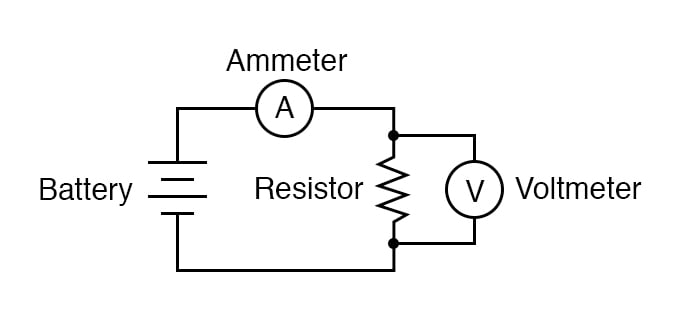ILLUSTRATION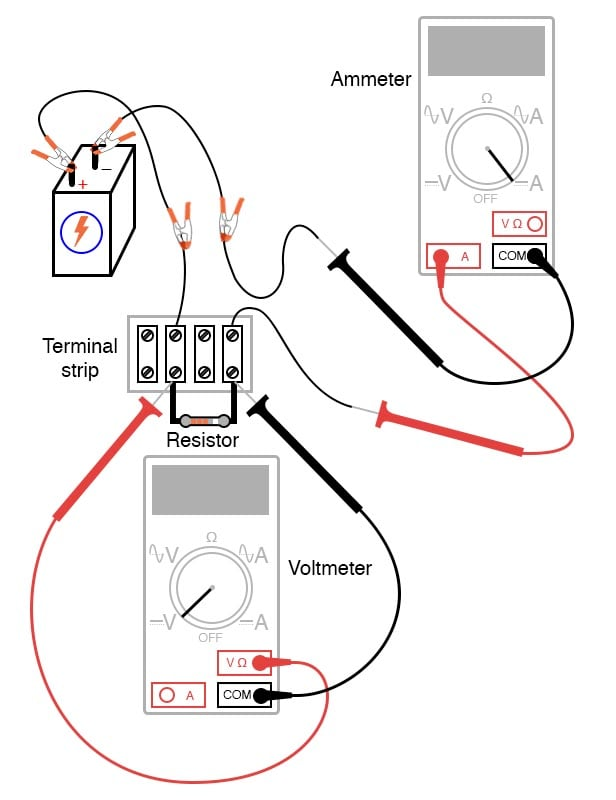INSTRUCTIONS

Select a resistor from the assortment, and measure its resistance with your multimeter set to the appropriate resistance range.

Be sure not to hold the resistor terminals when measuring resistance, or else your hand-to-hand body resistance will influence the measurement!

Record this resistance value for future use. Build a one-battery, one-resistor circuit.

A terminal strip is shown in the illustration, but any form of circuit construction is okay.

Set your multimeter to the appropriate voltage range and measure voltage across the resistor as it is being powered by the battery.

Record this voltage value along with the resistance value previously measured.

Set your multimeter to the highest current range available. Break the circuit and connect the ammeter within that break, so it becomes a part of the circuit, in series with the battery and resistor.

Select the best current range: whichever one gives the strongest meter indication without over-ranging the meter.

If your multimeter is autoranging, of course, you need not bother with setting ranges.

Record this current value along with the resistance and voltage values previously recorded.

Taking the measured figures for voltage and resistance, use the Ohm’s Law equation to calculate circuit current. Compare this calculated figure with the measured figure for circuit current: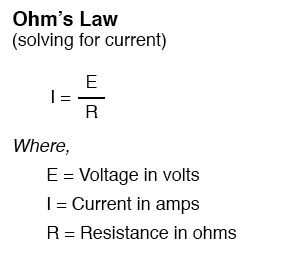Taking the measured figures for voltage and current, use the Ohm’s Law equation to calculate circuit resistance.

Compare this calculated figure with the measured figure for circuit resistance: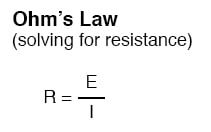Finally, taking the measured figures for resistance and current, use the Ohm’s Law equation to calculate circuit voltage.

Compare this calculated figure with the measured figure for circuit voltage: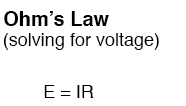There should be close agreement between all measured and all calculated figures.

Any differences in respective quantities of voltage, current, or resistance are most likely due to meter inaccuracies.

These differences should be rather small, no more than several percent. Some meters, of course, are more accurate than others!

Substitute different resistors in the circuit and re-take all resistance, voltage, and current measurements.

Re-calculate these figures and check for agreement with the experimental data (measured quantities).

Also note the simple mathematical relationship between changes in resistor value and changes in circuit current.

Voltage should remain approximately the same for any resistor size inserted into the circuit, because it is the nature of a battery to maintain voltage at a constant level.

Be sure to check out our Ohm’s Law Calculator.

RELATED WORKSHEETS:

Published under the terms and conditions of the Design Science License